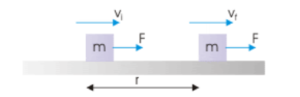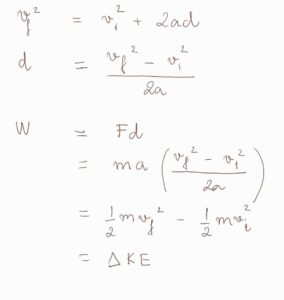Blog / Work Kinetic Energy Theorem

### Work Kinetic Energy Theorem

Written by Chau Pham on Feb 26, 2020

The work-energy theorem states that the work done by all forces acting on a particle equals the change in the particle’s kinetic energy.

A force does work on the block and set it in motion. The kinetic energy of the block (the energy that it possesses due to its motion) increases as a result by the amount of work.Vi and Vf are the speeds of the particle before and after the application of force, and m is the particle’s mass.With the knowledge of this relationship, try the energy approach first before applying kinematics when solving a problem,as energy approach is much easier

### KEY TAKEAWAYS

Key Points:

• The work W done by the net force on a particle equals the change in the particle’s kinetic energy KE
• The work-energy theorem can be derived from Newton’s second law.
• Prioritize energy approach to kinematics in problem solving.

Key Terms:

• Kinetic energy of an object is the energy that it possesses due to its motion

Billing Information